# Tree Machine

## Generate virtual trees with p5@polytroper

Guess what friends, today we’re making trees. That’s right, NATURE.

Here’s my tree, for your perusal: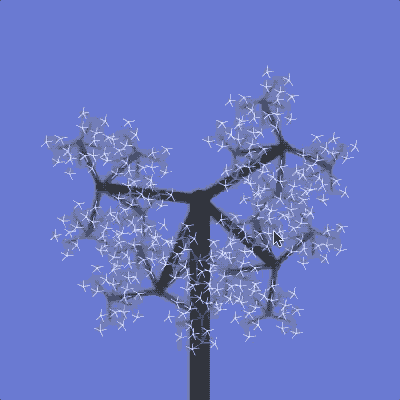How can a whole tree fit inside a computer, you may ask? The answer is simple: recursion.

## Drawing a line

First we’ll draw a line using p5. We want it to look like this: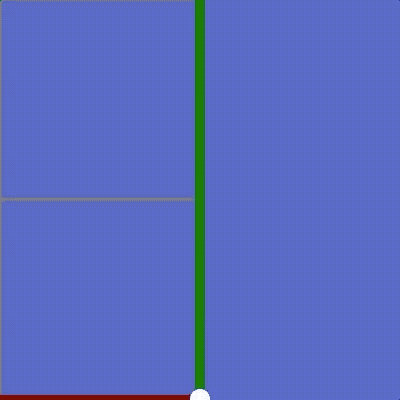Your file should look like this to start:

``````<html>
<title>Tree Machine</title>

<!-- Import p5 -->
<script src="https://cdn.jsdelivr.net/npm/p5@1.0.0/lib/p5.min.js"></script>

<script>
function setup() {
// Start off by creating a canvas to draw on
createCanvas(400, 400)
}

function draw() {
// Draw a black background
background(0)
}
</script>
</html>
``````

Like almost every p5 application, we start with a `setup` and `draw` function.

In `setup` we create a canvas for drawing. In `draw` we create a black background.

Let’s add a line on top of that background. Add these three new lines to your `draw` function, after `background`:

``````function draw() {
// Draw a black background
background(0)

// Set line color to white
stroke(255)

// Set thickness to 20
strokeWeight(20)

// Draw a line from (middle, bottom) to (middle, middle)
line(200, 400, 200, 200)
}
``````

Now if you hit Run you should see a white line going from the bottom of the canvas to the center.

Look at the last line of code: `line(200, 400, 200, 200)`

This is one way to draw a line—I specified where on the canvas to start, and where on the canvas to end.

However, there is another way to think about this. A trick, if you will. A trick that makes this whole “tree” drawing process much easier.

Instead of specifying where to draw on the canvas, we can actually translate (move), rotate, and scale (zoom) the canvas itself.

``````function draw() {
// Draw a black background
background(0)

// Set line color to white
stroke(255)

// Move to middle-bottom of canvas
translate(200, 400)

// Turn canvas 180°
rotate(Math.PI)

// Zoom way into the canvas
scale(200)

// Set thickness to a much smaller, zoomed-in value
strokeWeight(0.1)

// Draw a line from (0, 0) to (0, 1)
line(0, 0, 0, 1)
}
``````

Now look at that last line again.

Instead of `line(200, 400, 200, 200)` It now says `line(0, 0, 0, 1)`

This is because instead of saying exactly where on the canvas we should draw our line, we first used…

`translate(200, 400)` `rotate(Math.PI)` `scale(200)`

To move the canvas itself.

If we didn’t translate/rotate/scale the plane first, `line(0, 0, 0, 1)` would create a line from (0, 0) to (0, 1).

But instead we get a line from (200, 400) to (200, 200) because first we translated the canvas to (200, 400), rotated the canvas by pi (180°), and scaled the canvas by 200.

Now if you run your page you should get a simple white line, just as before.

Your whole script should look like this:

``````<script>
function setup() {
// Start off by creating a canvas to draw on
createCanvas(400, 400)
}

function draw() {
// Draw a black background
background(0)

// Set line color to white
stroke(255)

// Move to middle-bottom of canvas
translate(width / 2, height)

// Turn canvas 180°
rotate(Math.PI)

// Zoom way into the canvas
scale(200)

// Set thickness to a much smaller, zoomed-in value
strokeWeight(0.1)

// Draw a line from (0, 0) to (0, 1)
line(0, 0, 0, 1)
}
</script>
``````

## Depth

Now we’ll draw a line at the end of our line, like this: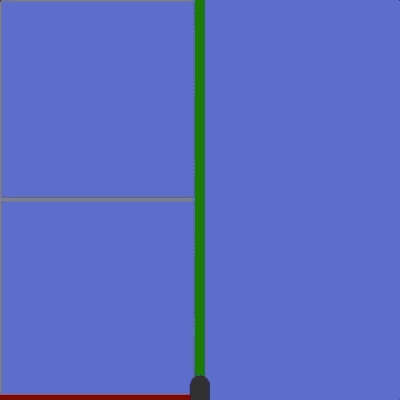This is where recursion comes in. Recursion is when one function calls itself.

When we draw a branch, we want to draw another branch at the end of that branch. The number of times we repeat that process is called the depth of our tree.

First, add a variable at the top of your script for `depth`. We’ll set it to 5 for now:

``````<script>
// How many levels of branches we will draw
var depth = 5

// ...the rest of the script
</script>
``````

Then, just after that, we’ll add a function called `branch` which draws each branch for us.

``````var depth = 5

function branch(d) {
// Draw a line from (0, 0) to (0, 1)
line(0, 0, 0, 1)

// If there are any more branch levels to be drawn...
if (d > 1) {
// Move the canvas to (0, 1), the end of our new line
translate(0, 1)

// Zoom twice as far into the canvas
scale(0.5)

// Recurse for the next branch!
branch(d - 1)
}
}

// ...the rest of the script
``````

Let’s break down what `branch` is doing.

1. First it draws a line
2. If any more lines need to be drawn…
1. Translate/scale (move/zoom) do the end of that line
2. Repeat for the next “depth” level (depth-1)

That last step—where we call `branch` again—is recursion. The function repeats itself, one level down.

If we didn’t have the `d-1` part, then the function would never know to stop “recursing”. That’s why we pass in a number for “depth” (`d`), and subtract 1 for each level; once we hit level 1, we know we can stop.

Finally, let’s change the last line of our `draw` function to use `branch(depth)` instead of `line(0, 0, 0, 1)`:

``````function draw() {
// Draw a black background
background(0)

// Set line color to white
stroke(255)

// Move to middle-bottom of canvas
translate(200, 400)

// Turn canvas 180°
rotate(Math.PI)

// Zoom way into the canvas
scale(200)

// Set thickness to a much smaller, zoomed-in value
strokeWeight(0.1)

// Start drawing branches!
branch(depth)
}
``````

Now if you hit Run, you should get a bunch of lines stacked on each other.

At this point, here’s what your whole script should be:

``````<script>
// How many levels of branches we will draw
var depth = 5

function branch(d) {
// Draw a line from (0, 0) to (0, 1)
line(0, 0, 0, 1)

// If there are any more branch levels to be drawn...
if (d > 1) {
// Move the canvas to (0, 1), the end of our new line
translate(0, 1)

// Zoom twice as far into the canvas
scale(0.5)

// Recurse for the next branch!
branch(d - 1)
}
}

function setup() {
// Start off by creating a canvas to draw on
createCanvas(400, 400)
}

function draw() {
// Draw a black background
background(0)

// Set line color to white
stroke(255)

// Move to middle-bottom of canvas
translate(200, 400)

// Turn canvas 180°
rotate(Math.PI)

// Zoom way into the canvas
scale(200)

// Set thickness to a much smaller, zoomed-in value
strokeWeight(0.1)

// Start drawing branches!
branch(depth)
}
</script>
``````

## Curl

Now we’ll rotate the canvas a little bit for each line. Watch what happens: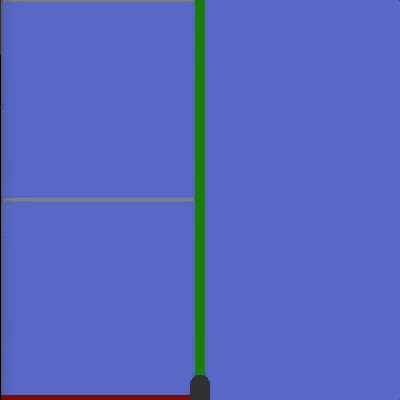For each new branch, the canvas rotates by a certain angle. We’ll call this angle the “curl”. Let’s create a variable for it at the top of our script, after `depth`:

``````<script>
// How many levels of branches we will draw
var depth = 5

// The angle each new branch level rotates by (branches spin left/right)
var curl = Math.PI / 3

// the rest of the script...
</script>
``````

Now in our `branch` function, let’s add a line to `rotate` the canvas by `curl` for each new branch.

I put this new line between `translate` and `scale`:

``````function branch(d) {
// Draw a line from (0, 0) to (0, 1)
line(0, 0, 0, 1)

// If there are any more branch levels to be drawn...
if (d > 1) {
// Move the canvas to (0, 1), the end of our new line
translate(0, 1)

// Rotate the canvas for each new branch
rotate(curl)

// Zoom twice as far into the canvas
scale(0.5)

// Recurse for the next branch!
branch(d - 1)
}
}
``````

Now if you hit Run, you should see your branches curl up!

Now your entire script should look like this:

``````<script>
// How many levels of branches we will draw
var depth = 5

// The angle each new branch level rotates by (branches spin left/right)
var curl = Math.PI / 3

function branch(d) {
// Draw a line from (0, 0) to (0, 1)
line(0, 0, 0, 1)

// If there are any more branch levels to be drawn...
if (d > 1) {
// Move the canvas to (0, 1), the end of our new line
translate(0, 1)

// Rotate the canvas for each new branch
rotate(curl)

// Zoom twice as far into the canvas
scale(0.5)

// Recurse for the next branch!
branch(d - 1)
}
}

function setup() {
// Start off by creating a canvas to draw on
createCanvas(400, 400)
}

function draw() {
// Draw a black background
background(0)

// Set line color to white
stroke(255)

// Move to middle-bottom of canvas
translate(200, 400)

// Turn canvas 180°
rotate(Math.PI)

// Zoom way into the canvas
scale(200)

// Set thickness to a much smaller, zoomed-in value
strokeWeight(0.1)

// Start drawing branches!
branch(depth)
}
</script>
``````

## Wave

Now let’s make our branches dance: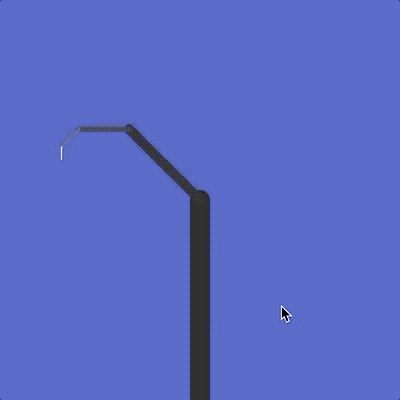All I am doing here is using the `mouseX` variable to change the `curl` variable.

Add this line to the top of your `draw` function:

``````function draw() {
// Set the curl angle with the mouse X position
curl = Math.PI * ((mouseX / width) * 2 - 1)

// the rest of the function...
}
``````

Let’s stop for a sec and break down what this line does: `curl = Math.PI*(mouseX/width*2-1)`

• `mouseX/width` gives us the fraction of the mouse X position compared to the width canvas. This will be 0 on the left side of the canvas, and 1 on the right side.

• `(mouseX/width*2-1)` will be -1 on the left side of the canvas, and 1 on the right side.

• `Math.PI*(mouseX/width*2-1)` will be -pi on the left side of the canvas, and +pi on the right side.

This way, our tree will curl from a rotation of -pi (-180°) in one direction to +pi (180°) in the other direction.

This should be your full script now:

``````<script>
// How many levels of branches we will draw
var depth = 5

// The angle each new branch level rotates by (branches spin left/right)
var curl = Math.PI / 3

function branch(d) {
// Draw a line from (0, 0) to (0, 1)
line(0, 0, 0, 1)

// If there are any more branch levels to be drawn...
if (d > 1) {
// Move the canvas to (0, 1), the end of our new line
translate(0, 1)

// Rotate the canvas for each new branch
rotate(curl)

// Zoom twice as far into the canvas
scale(0.5)

// Recurse for the next branch!
branch(d - 1)
}
}

function setup() {
// Start off by creating a canvas to draw on
createCanvas(400, 400)
}

function draw() {
// Set the curl angle with the mouse X position
curl = Math.PI * ((mouseX / width) * 2 - 1)

// Draw a black background
background(0)

// Set line color to white
stroke(255)

// Move to middle-bottom of canvas
translate(200, 400)

// Turn canvas 180°
rotate(Math.PI)

// Zoom way into the canvas
scale(200)

// Set thickness to a much smaller, zoomed-in value
strokeWeight(0.1)

// Start drawing branches!
branch(depth)
}
</script>
``````

Let’s add more branches to our tree: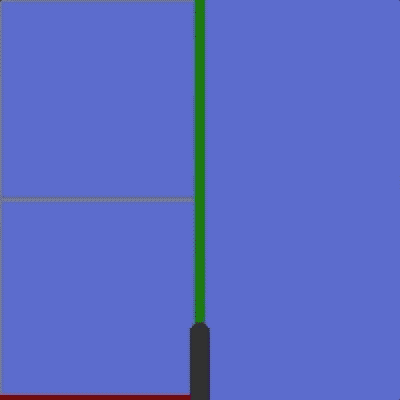Right now, each branch creates 1 new branch. We need each branch to create 2 new branches.

The number of new branches each branch creates is called the breadth of the tree. We’ll make it 2 for now.

Each branch will fan out from the last branch at a particular angle. We’ll call this angle the spread of the tree.

Let’s create two new variables at the top of our script for `breadth` and `spread`:

``````<script>
// How many levels of branches we will draw
var depth = 5

// The angle each new branch level rotates by (branches spin left/right)
var curl = Math.PI / 3

// How many branches each new branch will create

// The angle between each sub-branch (branches fan out/in)
var spread = Math.PI / 3

// the rest of the script...
</script>
``````

Now we need to do a few things to our `branch` function to make multiple branches happen.

Instead of one call to `branch(d-1)`, we need a loop that makes multiple calls to `branch(d-1)` and rotates the canvas by `spread` each time.

Replace that `branch(d-1)` line with a `for` loop, like this:

``````function branch(d) {
// Draw a line from (0, 0) to (0, 1)
line(0, 0, 0, 1)

// If there are any more branch levels to be drawn...
if (d > 1) {
// Move the canvas to (0, 1), the end of our new line
translate(0, 1)

// Rotate the canvas for each new branch
rotate(curl)

// Zoom twice as far into the canvas
scale(0.5)

for (var i = 0; i < breadth; i++) {
// Recurse for the next branch!
branch(d - 1)

// Rotate for the next branch!
}
}
}
``````

Now if you hit Run… it won’t quite work. You should see multiple branches, but they’re not rotated or positioned correctly. What’s going on here?

Watch that gif at the top of this chapter. Watch how the tree grows:

1. First it draws every level of one branch.
2. Then it “backs up” to draw any remaining branches, one level up.
3. Repeat—always drawing as many levels as possible before “backing up”

This is called depth-first drawing—as opposed to breadth-first drawing, where each level would fill in completely before starting on the next level.

Because we have to “back up” each time we finish drawing a branch, we actually need to undo everything we did to the canvas for that branch:

• Undo the `translate`
• Undo the `scale`
• Undo the `rotate` (for both `curl` and `spread`)

You can see this happen in the gif above. Anytime that reference grid gets bigger, it means the bottom level of a branch is complete and the tree has “backed up” at least one level.

To make this happen, add these four new lines just after that `for` loop:

``````for (var i = 0; i < breadth; i++) {
// Recurse for the next branch!
branch(d - 1)

// Rotate for the next branch!
}

// Undo the rotations we applied for each "child" branch

// Undo the curl rotation we applied to this branch
rotate(-curl)

// Zoom back out from the canvas
scale(2)

// Move back to the start of the line we drew with line(0, 0, 0, 1)
translate(0, -1)
``````

Now your script should look like this:

``````<script>
// How many levels of branches we will draw
var depth = 5

// The angle each new branch level rotates by (branches spin left/right)
var curl = Math.PI / 3

// How many branches each new branch will create

// The angle between each sub-branch (branches fan out/in)
var spread = Math.PI / 3

function branch(d) {
// Draw a line from (0, 0) to (0, 1)
line(0, 0, 0, 1)

// If there are any more branch levels to be drawn...
if (d > 1) {
// Move the canvas to (0, 1), the end of our new line
translate(0, 1)

// Rotate the canvas for each new branch
rotate(curl)

// Zoom twice as far into the canvas
scale(0.5)

for (var i = 0; i < breadth; i++) {
// Recurse for the next branch!
branch(d - 1)

// Rotate for the next branch!
}

// Undo the rotations we applied for each "child" branch

// Undo the curl rotation we applied to this branch
rotate(-curl)

// Zoom back out from the canvas
scale(2)

// Move back to the start of the line we drew with line(0, 0, 0, 1)
translate(0, -1)
}
}

function setup() {
// Start off by creating a canvas to draw on
createCanvas(400, 400)
}

function draw() {
// Set the curl angle with the mouse X position
curl = Math.PI * ((mouseX / width) * 2 - 1)

// Draw a black background
background(0)

// Set line color to white
stroke(255)

// Move to middle-bottom of canvas
translate(200, 400)

// Turn canvas 180°
rotate(Math.PI)

// Zoom way into the canvas
scale(200)

// Set thickness to a much smaller, zoomed-in value
strokeWeight(0.1)

// Start drawing branches!
branch(depth)
}
</script>
``````

Finally, let’s control that `spread` variable with the mouse: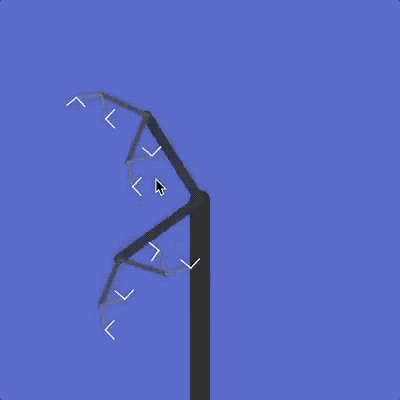This works just like the `curl` variable. Add another line at the top of your `draw` function, just after the line where you set `curl` with the mouse:

``````function draw() {
// Set the curl angle with the mouse X position
curl = Math.PI * ((mouseX / width) * 2 - 1)

// Set the spread angle with the mouse Y position
spread = Math.PI * ((mouseY / height) * 2 - 1)

// the rest of the function...
}
``````

That’s it! If you hit Run, you should be able to make your tree dance with your mouse.

Your final script should look like this:

``````<script>
// How many levels of branches we will draw
var depth = 5

// The angle each new branch level rotates by (branches spin left/right)
var curl = Math.PI / 3

// How many branches each new branch will create

// The angle between each sub-branch (branches fan out/in)
var spread = Math.PI / 3

function branch(d) {
// Draw a line from (0, 0) to (0, 1)
line(0, 0, 0, 1)

// If there are any more branch levels to be drawn...
if (d > 1) {
// Move the canvas to (0, 1), the end of our new line
translate(0, 1)

// Rotate the canvas for each new branch
rotate(curl)

// Zoom twice as far into the canvas
scale(0.5)

for (var i = 0; i < breadth; i++) {
// Recurse for the next branch!
branch(d - 1)

// Rotate for the next branch!
}

// Undo the rotations we applied for each "child" branch

// Undo the curl rotation we applied to this branch
rotate(-curl)

// Zoom back out from the canvas
scale(2)

// Move back to the start of the line we drew with line(0, 0, 0, 1)
translate(0, -1)
}
}

function setup() {
// Start off by creating a canvas to draw on
createCanvas(400, 400)
}

function draw() {
// Set the curl angle with the mouse X position
curl = Math.PI * ((mouseX / width) * 2 - 1)

// Set the spread angle with the mouse Y position
spread = Math.PI * ((mouseY / height) * 2 - 1)

// Draw a black background
background(0)

// Set line color to white
stroke(255)

// Move to middle-bottom of canvas
translate(200, 400)

// Turn canvas 180°
rotate(Math.PI)

// Zoom way into the canvas
scale(200)

// Set thickness to a much smaller, zoomed-in value
strokeWeight(0.1)

// Start drawing branches!
branch(depth)
}
</script>
``````

## Epilogue

Trees show up everywhere in programming.

The memory in your computer, the pages on every website, and every HTML element on every page are all arranged in trees.

It’s kind of remarkable that when you actually draw a tree, it looks so much like… a tree: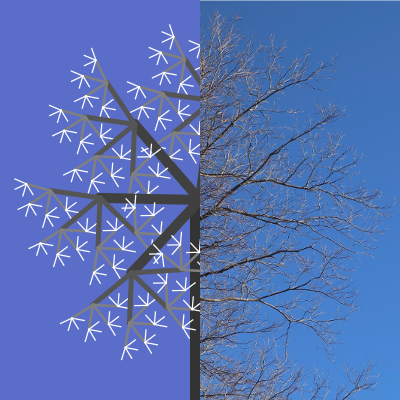Using a 3D version of the technique we just learned, you can generate some freakishly lifelike trees: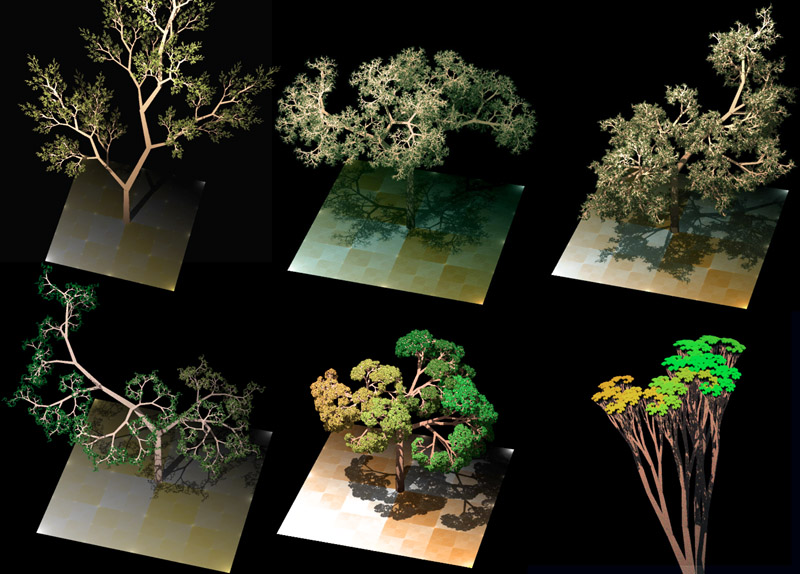And game developers take those techniques even further to generate realistic 3D models of trees: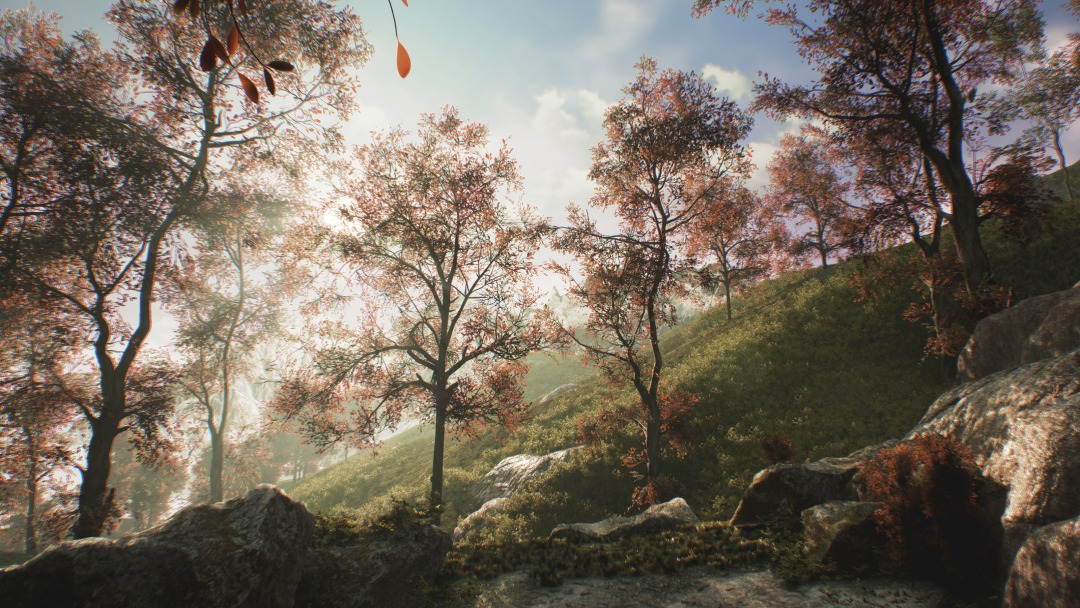Try messing with the numbers in your tree maker. You can get all kinds of cool effects: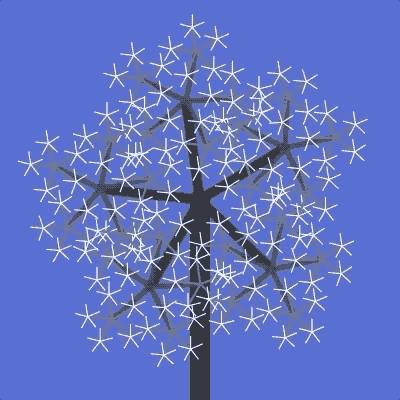See what you can come up with!